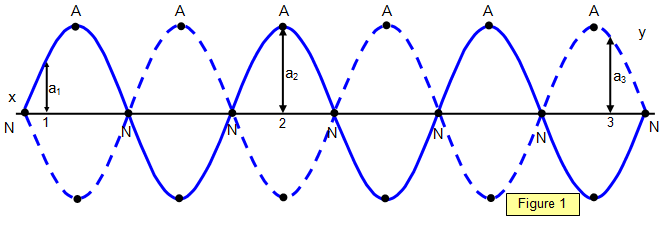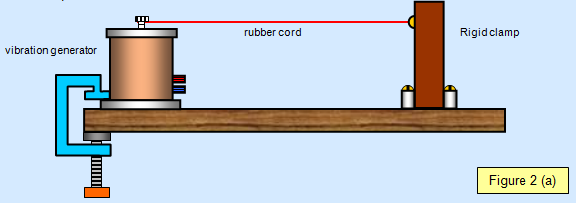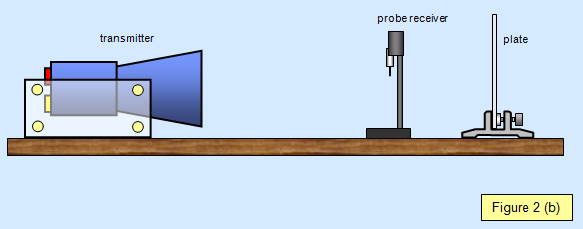Standing waves

A stationary or standing wave is one in which the amplitude varies from place to place along the wave. In a standing wave some points along the wave are stationary, while others vibrate with amplitudes up to the maximum for that wave.Figure 1 is a diagram of a stationary wave. Note that there are places where the amplitude is zero and, halfway between, places where the amplitude is a maximum; these are known as nodes (labelled N) and antinodes (labelled A) respectively.
(See Figure 1)

A node is a place of zero amplitude
An antinode is a place of maximum amplitude

The amplitude at any point in space on a standing wave does not change with time. In a travelling (progressive) wave the amplitude at any given point in space changes as time passes. The time between maximum amplitude at any point is the period of the wave (1/frequency).The distance between successive nodes, and successive antinodes, is half a wavelength. (λ/2)

The amplitude of the points on a stationary wave varies along the wave. In Figure 1 the amplitude at point 1 is a1, that at point 2 is a2 and that at point 3 is a3. The displacement (y) at these points varies with time.

Any stationary wave can be formed by the addition of two travelling (progressive) waves moving in opposite directions.

A wave moving in one direction reflects at a barrier and interferes with the incoming wave.

Mathematical treatment of the formation of a standing wave from two travelling (progressive) waves

Consider two travelling waves 1 and 2. Let the displacements at time t and position x be y1 and y2.

y1 = a sin (ωt - kx) (say right- left)
y2 = a sin (ωt + kx) (say left- right)

Therefore:

y1 + y2 = a sin (wt - kx) + a sin (ωt + kx) = 2a sin(ωt).cos(kx) = Asin (wt)

Note that this expression is composed of two terms:
(a) sin (wt) - this shows a varying amplitude with time at a particular place.
(b) cos (kx) - this shows a varying amplitude with position at a particular time.

When x = 0, l/2 ... A is a maximum and we have an antinode;
When x = λ/4, 3l/4, 5l/4 ... A is a minimum and we have a node.

Notice that the maximum value of A is 2a.schoolphysics: Standing waves video

To see a video of standing waves please click on the video link.

Student investigation

The standing waves on a string may be studied using an experiment due to Melde and shown in Figure 2(a). A 0.5 m long rubber cord of 3 mm2 cross-section is clamped at one end, stretched to twice its length and fixed to a vibrator. The vibration generator is connected to a signal generator which can give a range of frequencies between 10 Hz and 100 Hz.

Observe the effects of slowly increasing the vibrator frequency from 10 Hz to 100 Hz and explain what you see. The experiment is best done in a darkened laboratory with the cord illuminated with a stroboscope.Standing waves may also be investigated using the 2.8 cm wavelength microwave equipment used by most schools (Figure 2(b)).

The transmitter is set up facing a vertical metal plate about 50 cm away. If a probe detector connected to a meter is moved along the line between transmitter and plate a series of nodes and antinodes can be found. The distance between successive nodes or antinodes is half the wavelength of the microwaves.A VERSION IN WORD IS AVAILABLE ON THE SCHOOLPHYSICS USB